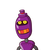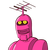# Q-1 express the following in exponential form :a) 2×2×a×a=b)5×5×7×7×7 =​

Q-1 express the following in exponential form :
a) 2×2×a×a
=
b)5×5×7×7×7
=​

### 2 thoughts on “Q-1 express the following in exponential form :<br />a) 2×2×a×a<br />=<br />b)5×5×7×7×7 <br />=​”

1.a)2²a²

b)5²×7³

Step-by-step explanation:

just see how many times the same number reapers and write down the powers

2.